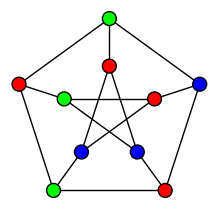CARI 2014 Extrait de la page de Nathann COHEN http://steinertriples.fr/ncohen/tut/LP_examples/

# Some LP Formulations(and their Sage code)

 Independent Set Maximum set of pairwise non-adjacent vertices in a graph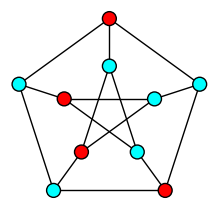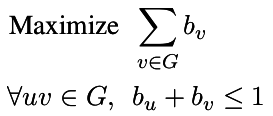``````p = MixedIntegerLinearProgram(maximization = True) b = p.new_variable(binary = True) p.set_objective( sum([b[v] for v in g]) ) for u,v in g.edges(labels = False): p.add_constraint( b[u] + b[v] <= 1 ) p.solve() b = p.get_values(b) print [v for v,i in b.items() if i] `````` Dominating Set Minimum set of vertices whose neighborhood is the whole graph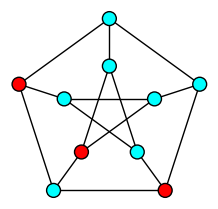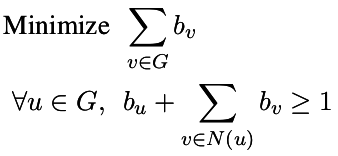Vertex Cover Minimum Set of vertices touching each edge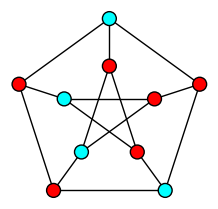Partition Partition a set of integers into two sets whose sum is equal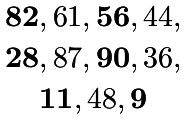Bipartite Set Partition the graph into two independent sets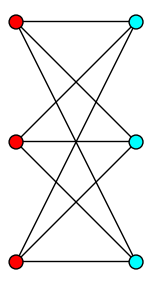Subset Sum Find a nonempty subset of integers with null sum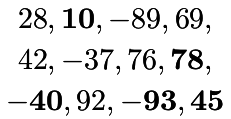Distances Compute the distance from vertex 0 to any other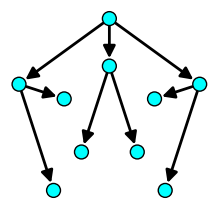Girth Size of the shortest cycle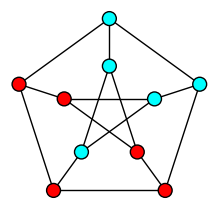Matching Maximum number of non-incident edges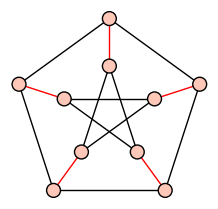Feedback Arc Set Minimum set of arcs hitting all circuits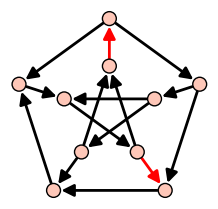Feedback Vertex Set Minimum set of vertices hitting all circuits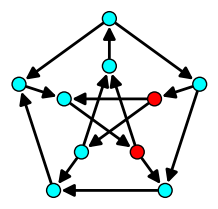Hamiltonian Cycle A cycle going through all vertices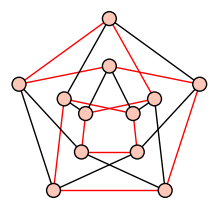3-coloration Partition a graph into three independent sets